# [Problem 3] A rope of length L circles around the origin O on XY plane as...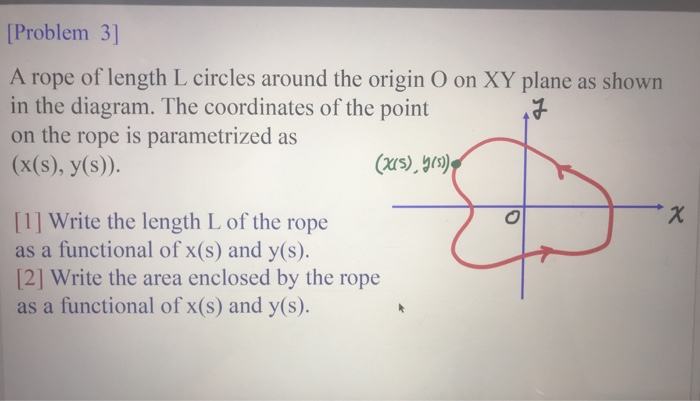[Problem 3] A rope of length L circles around the origin O on XY plane as shown in the diagram. The coordinates of the point on the rope is parametrized as (x(s), y(s)). (x(s), y(s) .x  Write the length L of the rope as a functional of x(s) and y(s).  Write the area enclosed by the rope as a functional of x(s) and y(s).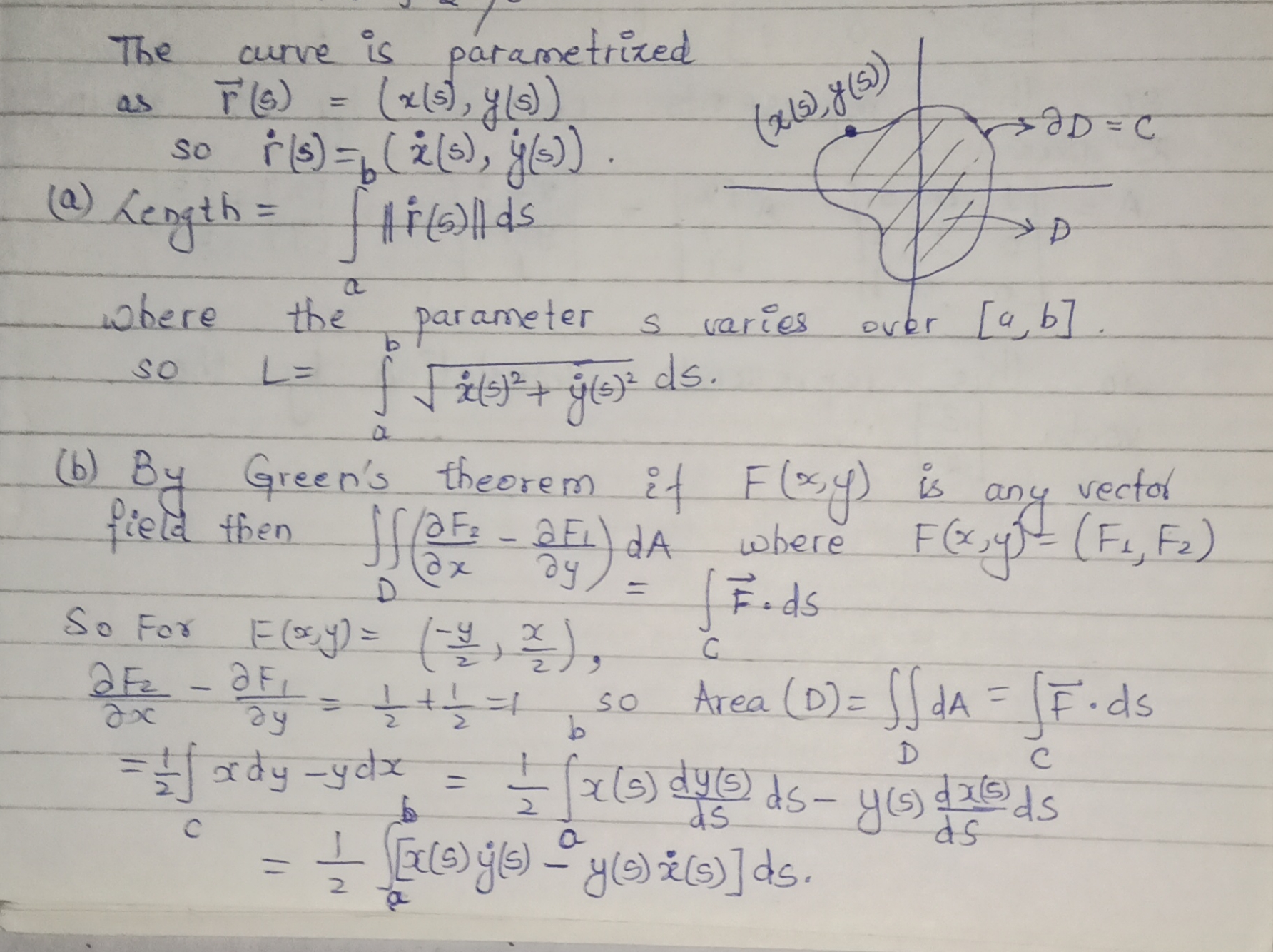##### Add Answer of: [Problem 3] A rope of length L circles around the origin O on XY plane as...
Similar Homework Help Questions
• ### On the xy-coordinate plane, point O is the origin

On the xy-coordinate plane, point O is the origin. Suppose vector OE=(3,8) and vector OF=(-2,-4). What ordered pair represents vector EF?I tried graphing them but they don't even end up in the same quadrant.Not sure what to do..

• ### In the xy-plane, line l passes through the origin and is perpendicular to the line 4x+y=k, where k is a constant

In the xy-plane, line l passes through the origin and is perpendicular to the line 4x+y=k, where k is a constant. If the two lines intersect at the point (t,t+1), what is the value of t?I tried drawing a picture of the problem described above, but I don't understand what I need to do to solve it.

• ### Question 3) The diagram is about the motion of an object in the xy plane: Overall the object starts at point A on t...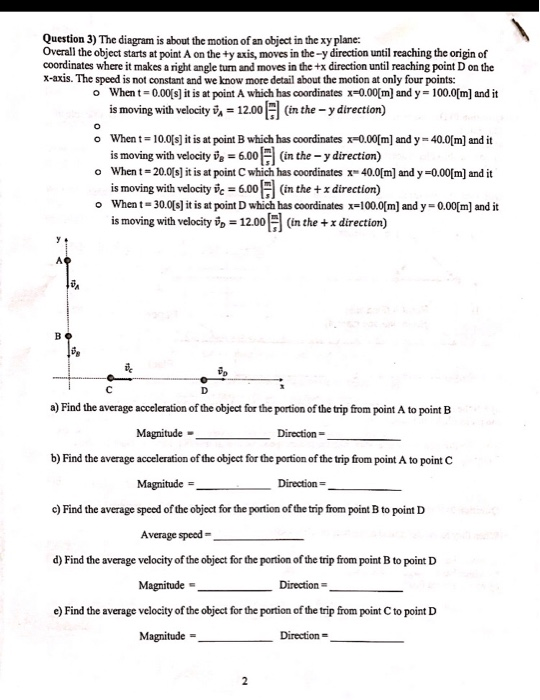Question 3) The diagram is about the motion of an object in the xy plane: Overall the object starts at point A on the +y axis, moves in the-y direction until reaching the origin of coordinates where it makes a right angle turn and moves in the +x direction until reaching point D on the x-axis. The speed is not constant and we know more detail about the motion at only four points: When t-0.00s] it is at point A...

• ### Please explain the solution 3. A massless rope of length l, attached toa fixed pivot at...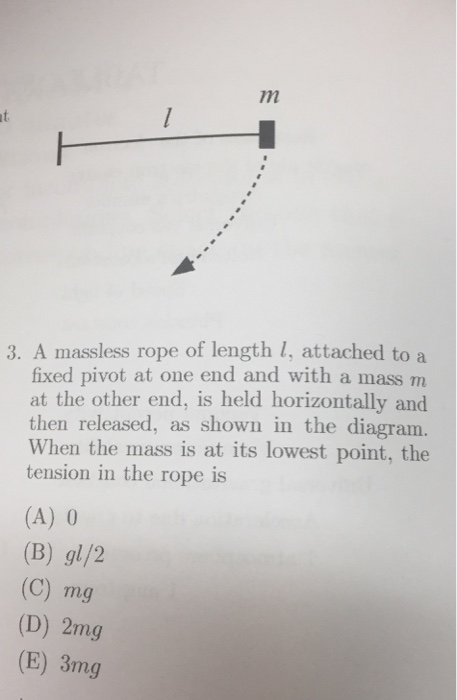Please explain the solution 3. A massless rope of length l, attached toa fixed pivot at one end and with a mass m at the other end, is held horizontally and then released, as shown in the diagram. When the mass is at its lowest point, the tension in the rope is (A) 0 (B) gl/2 (C) mg (D) 2mg (E) 3mg

• ### A point rotates about the origin in the xy plane at a constant radius of 0.102...

A point rotates about the origin in the xy plane at a constant radius of 0.102 m and angular velocity of 8.91 rad/s. As you know, the projections of this point on the x- and y-axes undergo simple harmonic motion. A. What is the amplitude of this motion? B. What is its frequency? C. What is its period?

• ### the moment about the xy-plane

Suppose E is the sold bounded by the paraboloid z=x^2+y^2-3 and above by the paraboloid z=9-2(x^2+y^2).a. Write down a triple integral in cylindrical coordinates for Mxy, the moment about the xy-plane, if the density is 1.b. Suppose the integral from part (a) yields Mxy = 56pi. Use this fact, symmetry, and the fact that the mass of the solid is m=24pi to write down the centroid for thesolid E.

• ### A point retates about the origin in the xy plane at a constant radius of 0.120...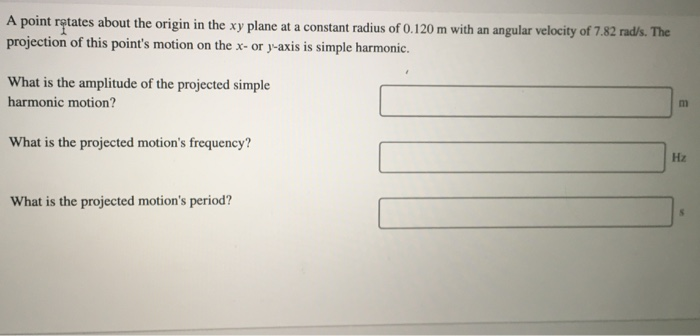A point retates about the origin in the xy plane at a constant radius of 0.120 m with an angular velocity of 7.82 rad/s. The projection of this point's motion on the x- or y-axis is simple harmonic. What is the amplitude of the projected simple harmonic motion? What is the projected motion's frequency? Нz What is the projected motion's period?

• ### Consider a rope with length l, mass per unit length ?, experiencing a gravitational acceleration g and hanging verticall...

Consider a rope with length l, mass per unit length ?, experiencing a gravitational acceleration g and hanging vertically as shown. (Figure 1) Let y refer to the height of a point P above the bottom of the rope.

• ### A person P, starting at the origin, moves in the direction of the positive x-axis, pulling...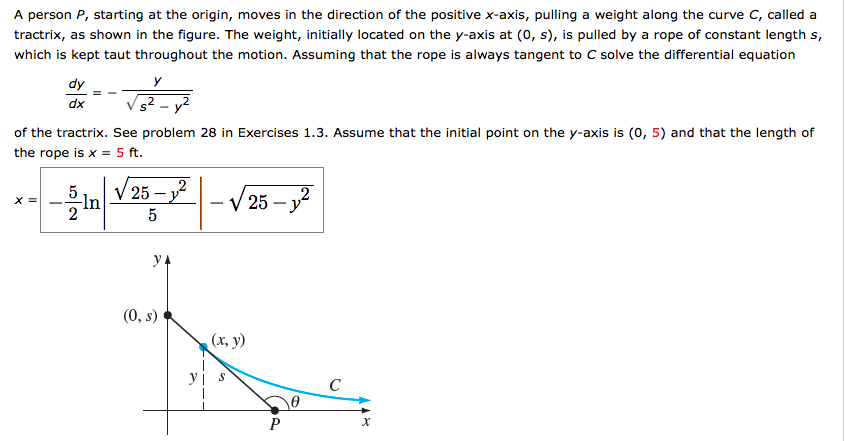A person P, starting at the origin, moves in the direction of the positive x-axis, pulling a weight along the curve C, called a shown in the figure. The weight, initially located on the y-axis at (0, s), is pulled by a rope of constant length s, tractrix, as which is kept taut throughout the motion. Assuming that the rope is always tangent to C solve the differential equation dy dx of the tractrix. See problem 28 in Exercises 1.3....

• ### 3. What point on the line y = 7 - 3x is closest to the origin?...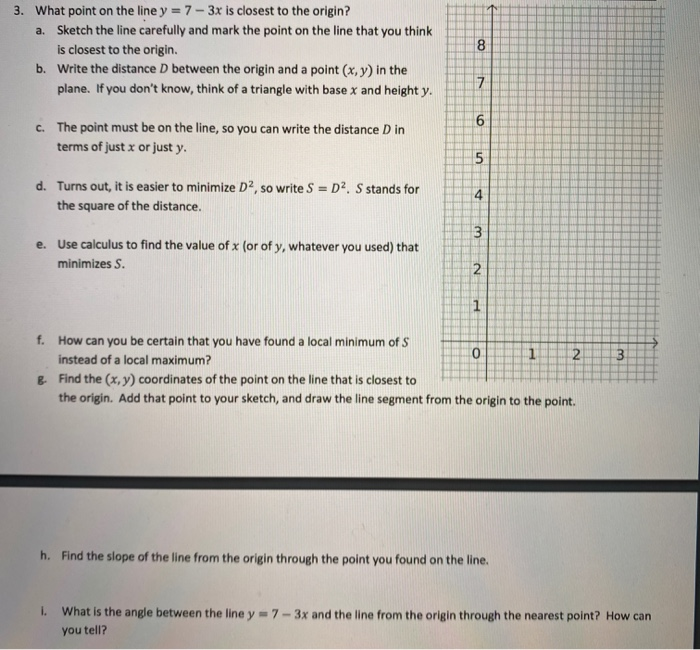3. What point on the line y = 7 - 3x is closest to the origin? a. Sketch the line carefully and mark the point on the line that you think is closest to the origin. b. Write the distance between the origin and a point (x,y) in the plane. If you don't know, think of a triangle with base x and height y. 8 7 6 c. The point must be on the line, so you can write the...

Free Homework App